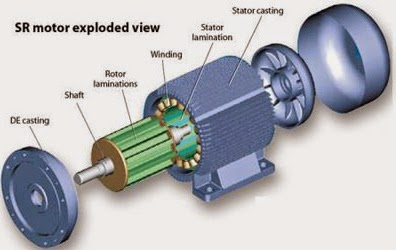## Minggu, 14 Desember 2014

### Characteristics And Work Principles of Switched Reluctance (SR) MotorCharacteristics And Work Principles of Switched Reluctance (SR) Motor (photo credit: machinedesign.com)

## SR Motor Characteristics

The Switched Reluctance (SR) motor is very different from the other polyphase machines described because both the stator and the rotor have salient poles. The motor can only be used in conjunction with its specific power converter and control, and consequently only overall characteristics are relevant.
The SR motor produces torque through the magnetic attraction which occurs between stator electromagnets and a corresponding set of salient poles formed on a simple rotor made only of ferromagnetic material.
The intuitively straight forward principle of torque production is easily visualized in the very simple reluctance motor illustrated, in cross section, in Figure 1.Figure 1 – Simple reluctance motor
If current is applied to the winding the rotor will turn until it reaches a position where it is aligned with the coils, at which point the inductance of the magnetic circuit is a minimum. The characteristic variation of inductance of a SR motor is shown in Figure 2.Figure 2 – Variation of inductance with rotor angle
If the machine is lightly magnetically loaded and a modest level of torque is produced, then the steel from which the rotor and stator are made of will behave magnetically in an approximately linear fashion.
That is, for a given number of turns on the windings, the magnetic flux of a phase varies approximately in proportion to the phase current. If linearity is assumed, then it can be shown that the torque produced as a function of angle θ is:
T = [i(dL / dθ)] / 2
Equation above shows that the torque is not dependent upon the direction of the current, but depends upon whether the current is applied when the inductance L is rising or falling with angular position.
The phase currents are always switched synchronously with the mechanical position of the rotor. At low speeds, the phases are energized over the entire region of rising inductance, and active current limiting is required from the controller. Torque is controlled by adjusting the magnitude of the phase current.SR motor – Exploded view
As speed increases, the rise and fall times (especially the latter) of the phase current occupy significant rotor angle, and it is usual to advance the turn-on and turn-off angles with respect to rotor position. The torque is now controlled by both the current limit level and by the switching angles, though current is usually used as the primary control variable. At high speeds, the rise and fall times occupy still greater rotor angles.

The current naturally self-limits and it is usual to control the torque using only the switching angles. The shape of the current waveform is greatly influenced by the high rate of change of inductance with respect to time.
By choosing appropriate switching angles and current levels, together with an appropriate electromagnetic design, the torque-speed characteristic of the switched reluctance drive can be tailored to suit the application. Furthermore, simply by changing the control parameter selection with torque and speed, a given machine design can be made to offer a choice of different characteristics.Figure 3 – Cross section of three-phase 6–4 SR motor
The simple single-phase machine in Figure 1 is capable of producing torque over only half of its electrical cycle. More demanding applications use higher pole numbers on the rotor and stator, with the stator poles wound and connected into multiple identical phases. Figure 3 illustrates the cross section of a three-phase 6–4 machine, diametrically opposed coils being connected together to form three- phase circuits denoted A, B and C.

The excitation of the phases is interleaved equally throughout the electrical period of the machine. This means that torque of the desired polarity can be produced continuously. The number of phases can in theory be increased without limit, but one to four phases are most common for commercial and industrial applications.
Many different combinations of pole count are possible. It is sometimes beneficial to use more than one stator pole pair per phase, so that, for example, the 12–8 pole structure is commonly used for three-phase applications. Each phase circuit then comprises four stator coils connected and energized together. Increasing the phase number brings the advantage of smoother torque.